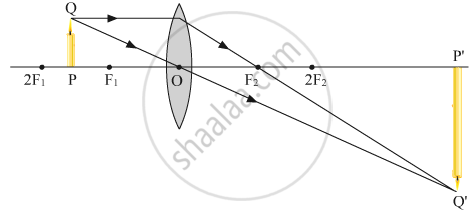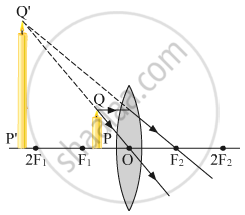Share

# Draw ray diagrams to show the formation of three times magnified (a) real, and (b) virtual image of an object by a converging lens. Mark the positions of O, F and 2F in each diagram. - CBSE Class 10 - Science

ConceptRefraction of Light Sign Convention for Spherical Lenses

#### Question

Draw ray diagrams to show the formation of three times magnified (a) real, and (b) virtual image of an object by a converging lens. Mark the positions of O, F and 2F in each diagram.

#### Solution

For real image: To get three times magnified image, the object is placed between the F1 and 2F1
The position of the object is set such that image distance is equal to the thrice of the object distance (v=3u).For virtual image: To get three times magnified image, the object is placed between the focus F1 and optical centre O.
The position of the object is set such that image distance is equal to the thrice of the object distance (i.e. v=3u).Is there an error in this question or solution?

#### Video TutorialsVIEW ALL 

Solution Draw ray diagrams to show the formation of three times magnified (a) real, and (b) virtual image of an object by a converging lens. Mark the positions of O, F and 2F in each diagram. Concept: Refraction of Light - Sign Convention for Spherical Lenses.
S